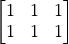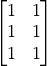# TensorFlow Hub 模型复用

## TF Hub 安装

pip install tensorflow-hub

## TF Hub 模型使用样例import tensorflow_hub as hub

outputs = hub_model(inputs)

hub.load(url) 就是把 TF Hub 的模型从网络下载和加载进来， hub_module 就是运行模型， outputs 即为输出

### 以 stylization 为例该模型的地址如下：

tfhub.dev，请大家转换域名到国内镜像 https://hub.tensorflow.google.cn/ ，模型下载地址也需要相应转换。import matplotlib.pyplot as plt
import numpy as np
import tensorflow as tf
import tensorflow_hub as hub

def crop_center(image):
"""Returns a cropped square image."""
shape = image.shape
new_shape = min(shape, shape)
offset_y = max(shape - shape, 0) // 2
offset_x = max(shape - shape, 0) // 2
image = tf.image.crop_to_bounding_box(image, offset_y, offset_x, new_shape, new_shape)
return image

# Load and convert to float32 numpy array, add batch dimension, and normalize to range [0, 1].
if img.max() > 1.0:
img = img / 255.
if len(img.shape) == 3:
img = tf.stack([img, img, img], axis=-1)
img = crop_center(img)
img = tf.image.resize(img, image_size, preserve_aspect_ratio=True)
return img

def show_image(image, title, save=False):
plt.imshow(image, aspect='equal')
plt.axis('off')
if save:
else:
plt.show()

content_image_path = "images/contentimg.jpeg"
style_image_path = "images/styleimg.jpeg"

show_image(content_image, "Content Image")
show_image(style_image, "Style Image")

# Stylize image.
outputs = hub_module(tf.constant(content_image), tf.constant(style_image))
stylized_image = outputs

show_image(stylized_image, "Stylized Image", True)

## TF Hub 模型retrain样例

需要进行二次训练。针对这种情况，TF Hub 提供了很方便的 Keras 接口 hub.KerasLayer(url) ，其可以封装在 Keras 的 Sequential 层状结构中，进而可以针对开发者的需求和数据进行再训练import tensorflow as tf
import tensorflow_hub as hub

num_classes = 10

# 使用 hub.KerasLayer 组件待训练模型
new_model = tf.keras.Sequential([
tf.keras.layers.Dense(num_classes, activation='softmax')
])
new_model.build([None, 299, 299, 3])

# 输出模型结构
new_model.summary()

# TensorFlow Datasets 数据集载入

pip install tensorflow-datasetsimport tensorflow as tf
import tensorflow_datasets as tfdsdataset = tfds.load("mnist", split=tfds.Split.TRAIN)
dataset = tfds.load("tf_flowers", split=tfds.Split.TRAIN, as_supervised=True)export TFDS_HTTPS_PROXY=http://代理服务器IP:端口

tfds.load 方法返回一个 tf.data.Dataset 对象。部分重要的参数如下：

• as_supervised ：若为 True，则根据数据集的特性，将数据集中的每行元素整理为有监督的二元组 (input, label) （即 “数据 + 标签”）形式，否则数据集中的每行元素为包含所有特征的字典。

• split：指定返回数据集的特定部分。若不指定，则返回整个数据集。一般有 tfds.Split.TRAIN （训练集）和 tfds.Split.TEST （测试集）选项。# 使用 TessorFlow Datasets 载入“tf_flowers”数据集
# 对 dataset 进行大小调整、打散和分批次操作
dataset = dataset.map(lambda img, label: (tf.image.resize(img, [224, 224]) / 255.0, label)) \
.shuffle(1024) \
.batch(32)
# 迭代数据
for images, labels in dataset:
# 对images和labels进行操作

# Swift for TensorFlow

## S4TF 环境配置

### 在 Colaboratory 中快速体验 Swift for TensorFlow

Google 的 Colaboratory 可以直接支持 Swift 语言的运行环境。可以 点此 直接打开一个空白的，具备 Swift 运行环境的 Colab Notebook ，这是立即体验 Swift for TensorFlow 的最方便的办法。

### 在 Docker 中快速体验 Swift for TensorFlow

• 获得一个 S4TS 的 Jupyter Notebook

在命令行中执行 nvidia-docker run -ti --rm -p 8888:8888 --cap-add SYS_PTRACE -v "\$(pwd)":/notebooks zixia/swift来启动 Jupyter ，然后根据提示的 URL ，打开浏览器访问即可。

• 获得一个已经安装好 S4TF 的 Swift REPL 环境

在命令行中执行 docker run -it --privileged --userns=host zixia/swift swift

## S4TF 基础使用import TensorFlow

// 声明两个Tensor
let x = Tensor<Float>()
let y = Tensor<Float>()

// 对两个 Tensor 做加法运算
let w = x + y

// 输出结果
print(w)

Swift 是动态强类型语言

### 在 Swift 中使用标准的 TensorFlow APIlet x = Tensor<BFloat16>(zeros: [32, 128])
let h1 = sigmoid(matmul(x, w1) + b1)
let h2 = tanh(matmul(h1, w1) + b1)
let h3 = softmax(matmul(h2, w1) + b1)let imageBatch = Dataset(elements: images)
let labelBatch = Dataset(elements: labels)
let zipped = zip(imageBatch, labelBatch).batched(8)

let imageBatch = Dataset(elements: images)
let labelBatch = Dataset(elements: labels)
for (image, label) in zip(imageBatch, labelBatch) {
let y = matmul(image, w) + b
let loss = (y - label).squared().mean()
print(loss)
}

matmul() 的别名： •

### 在 Swift 中直接加载 Python 语言库import Python

let np = Python.import("numpy")
let x = np.array([[1, 2], [3, 4]])
let y = np.array([11, 12])
print(x.dot(y))import Glibc
let x = malloc(18)
memcpy(x, "memcpy from Glibc", 18)
free(x)
Swift 也快成直接调用系统函数库。比如下面的代码例子展示了我们可以在 Swift 中直接加载 Glibc 的动态库，然后调用系统底层的 malloc 和 memcpy 函数，对变量直接进行操作

### 语言原生支持自动微分@differentiable
func frac(x: Double) -> Double {
return 1/x
}

gradient(of: frac)(0.5)

Swift 使用 func 声明一个函数。在函数的参数中，变量名的冒号后面代表的是 “参数类型”；在函数参数和函数体（{}） 之前，还可以通过瘦箭头（->）来指定函数的 返回值类型。import TensorFlow
import Python
import Foundation

import MNISTstruct MLP: Layer {
// 定义模型的输入、输出数据类型
typealias Input = Tensor<Float>
typealias Output = Tensor<Float>

// 定义 flatten 层，将二维矩阵展开为一个一维数组
var flatten = Flatten<Float>()
// 定义全连接层，输入为 784 个神经元，输出为 10 个神经元
var dense = Dense<Float>(inputSize: 784, outputSize: 10)

@differentiable
public func callAsFunction(_ input: Input) -> Output {
// 模型运行时，将 input 顺序传递给：flatten 和 dense 层，将结果返回作为模型输出。
return input.sequenced(through: flatten, dense)
}
}

callAsFunction(_:) 中可以通过类似 Keras 的 Sequential 的方法进行定义：input.sequences(through: layer1, layer2, ...) 将输入和所有的后续处理层 layer1layer2, … 等衔接起来。

Swift 中，每个函数参数都有一个 参数标签 (Argument Label) 以及一个 参数名称(Parameter Name)。 参数标签 主要应用在调用函数的情况，使得函数的实参与真实命名相关联，更加容易理解实参的意义。同时因为有 参数标签 的存在，实在的顺序是可以随意改变的。var model = MLP()

let mnist = MNIST()
let ((trainImages, trainLabels), (testImages, testLabels)) = mnist.loadData()

let imageBatch = Dataset(elements: trainImages).batched(32)
let labelBatch = Dataset(elements: trainLabels).batched(32)for (X, y) in zip(imageBatch, labelBatch) {
let logits = model(X)
return softmaxCrossEntropy(logits: logits, labels: y)
}

// Update parameters by optimizer
}

Swift 的闭包函数声明为：{ (parameters) -> return type in statements }，其中：parameters 为闭包接受的参数，return type 为闭包运行完毕的返回值类型，statements 为闭包内的运行代码。let logits = model(testImages)
let acc = mnist.getAccuracy(y: testLabels, logits: logits)

print("Test Accuracy: \(acc)" )

# TensorFlow in Julia

## TensorFlow.jl 环境配置

### 在 julia 包管理器中安装 TensorFlow.jl

using pkg
Pkg.add("TensorFlow")

## TensorFlow.jl 基础使用

using TensorFlow

# 定义一个 Session
sess = TensorFlow.Session() # 定义一个常量和变量 x = TensorFlow.constant() y = TensorFlow.Variable() # 定义一个计算 w = x + y # 执行计算过程 run(sess, TensorFlow.global_variables_initializer()) res = run(sess, w) # 输出结果 println(res)

### MNIST 数字分类# 使用自带例子中的 mnist_loader.jl 加载数据

# 定义一个 Session
using TensorFlow
sess = Session()

# 构建 softmax 回归模型
x = placeholder(Float32)
y_ = placeholder(Float32)
W = Variable(zeros(Float32, 784, 10))
b = Variable(zeros(Float32, 10))

run(sess, global_variables_initializer())

# 预测类和损失函数
y = nn.softmax(x*W + b)
cross_entropy = reduce_mean(-reduce_sum(y_ .* log(y), axis=))

# 开始训练模型
for i in 1:1000
run(sess, train_step, Dict(x=>batch, y_=>batch))
end

# 查看结果并评估模型
correct_prediction = indmax(y, 2) .== indmax(y_, 2)
accuracy=reduce_mean(cast(correct_prediction, Float32))

println(run(sess, accuracy, Dict(x=>testx, y_=>testy)))
MNIST 数字分类

# 图执行模式下的 TensorFlow

## TensorFlow 1+1

TensorFlow 的图执行模式是一个符号式的（基于计算图的）计算框架。简而言之，如果你需要进行一系列计算，则需要依次进行如下两步：

• 建立一个“计算图”，这个图描述了如何将输入数据通过一系列计算而得到输出；

• 建立一个会话，并在会话中与计算图进行交互，即向计算图传入计算所需的数据，并从计算图中获取结果。

### 使用计算图与会话进行基本运算import tensorflow.compat.v1 as tf
tf.disable_eager_execution()

# 以下三行定义了一个简单的“计算图”
a = tf.constant(1)  # 定义一个常量张量（Tensor）
b = tf.constant(1)
c = a + b  # 等价于 c = tf.add(a, b)，c是张量a和张量b通过 tf.add 这一操作（Operation）所形成的新张量

sess = tf.Session()     # 实例化一个会话（Session）
c_ = sess.run(c)        # 通过会话的 run() 方法对计算图里的节点（张量）进行实际的计算
print(c_)import tensorflow as tf

@tf.function
def graph():
a = tf.constant(1)  # 定义一个常量张量（Tensor）
b = tf.constant(1)
c = a + b
return c

c_ = graph()
print(c_.numpy())

### 占位符（Placeholder）与 feed_dictimport tensorflow.compat.v1 as tf
tf.disable_eager_execution()

a = tf.placeholder(dtype=tf.int32)  # 定义一个占位符Tensor
b = tf.placeholder(dtype=tf.int32)
c = a + b

a_ = int(input("a = "))  # 从终端读入一个整数并放入变量a_
b_ = int(input("b = "))

sess = tf.Session()
c_ = sess.run(c, feed_dict={a: a_, b: b_})  # feed_dict参数传入为了计算c所需要的张量的值
print("a + b = %d" % c_)import tensorflow as tf

@tf.function
def graph(a, b):
c = a + b
return c

a_ = int(input("a = "))
b_ = int(input("b = "))
c_ = graph(a_, b_)
print("a + b = %d" % c_)

• tf.placeholder() 相当于 tf.function 的函数参数；

• sess.run() 的 feed_dict 参数相当于给被 @tf.function 修饰的函数传值。

### 变量（Variable）import tensorflow.compat.v1 as tf
tf.disable_eager_execution()

a = tf.get_variable(name='a', shape=[])
initializer = tf.assign(a, 0.0)   # tf.assign(x, y)返回一个“将张量y的值赋给变量x”的操作
plus_one_op = tf.assign(a, a + 1.0)

sess = tf.Session()
sess.run(initializer)
for i in range(5):
sess.run(plus_one_op)       # 对变量a执行加一操作
print(sess.run(a))          # 输出此时变量a在当前会话的计算图中的值import tensorflow.compat.v1 as tf
tf.disable_eager_execution()

a = tf.get_variable(name='a', shape=[],
initializer=tf.zeros_initializer)   # 指定初始化器为全0初始化
plus_one_op = tf.assign(a, a + 1.0)

sess = tf.Session()
sess.run(tf.global_variables_initializer()) # 初始化所有变量
for i in range(5):
sess.run(plus_one_op)
print(sess.run(a))import tensorflow as tf

a = tf.Variable(0.0)

@tf.function
def plus_one_op():
a.assign(a + 1.0)
return a

for i in range(5):
plus_one_op()
print(a.numpy())

### 矩阵及张量计算import tensorflow.compat.v1 as tf
tf.disable_eager_execution()

A = tf.ones(shape=[2, 3])   # tf.ones(shape)定义了一个形状为shape的全1矩阵
B = tf.ones(shape=[3, 2])
C = tf.matmul(A, B)

sess = tf.Session()
C_ = sess.run(C)
print(C_)和## 基础示例：线性回归

ensorFlow 的图执行模式使用 符号式编程 来进行数值运算。import tensorflow as tf

# 定义数据流图
learning_rate_ = tf.placeholder(dtype=tf.float32)
X_ = tf.placeholder(dtype=tf.float32, shape=)
y_ = tf.placeholder(dtype=tf.float32, shape=)
a = tf.get_variable('a', dtype=tf.float32, shape=[], initializer=tf.zeros_initializer)
b = tf.get_variable('b', dtype=tf.float32, shape=[], initializer=tf.zeros_initializer)

y_pred = a * X_ + b
loss = tf.constant(0.5) * tf.reduce_sum(tf.square(y_pred - y_))

# 反向传播，手动计算变量（模型参数）的梯度
grad_a = tf.reduce_sum((y_pred - y_) * X_)

# 梯度下降法，手动更新参数
new_a = a - learning_rate_ * grad_a
new_b = b - learning_rate_ * grad_b
update_a = tf.assign(a, new_a)
update_b = tf.assign(b, new_b)

train_op = [update_a, update_b]
# 数据流图定义到此结束
# 注意，直到目前，我们都没有进行任何实质的数据计算，仅仅是定义了一个数据图

num_epoch = 10000
learning_rate = 1e-3
with tf.Session() as sess:
# 初始化变量a和b
tf.global_variables_initializer().run()
# 循环将数据送入上面建立的数据流图中进行计算和更新变量
for e in range(num_epoch):
sess.run(train_op, feed_dict={X_: X, y_: y, learning_rate_: learning_rate})
print(sess.run([a, b]))

### 自动求导机制

# 反向传播，手动计算变量（模型参数）的梯度
grad_a = tf.reduce_sum((y_pred - y_) * X_) grad_b = tf.reduce_sum(y_pred - y_) 

grad_a, grad_b = tf.gradients(loss, [a, b])

### 优化器

TensorFlow 在图执行模式下也附带有多种 优化器 （optimizer），可以将求导和梯度更新一并完成。我们可以将上节的代码

# 反向传播，手动计算变量（模型参数）的梯度
grad_a = tf.reduce_sum((y_pred - y_) * X_) grad_b = tf.reduce_sum(y_pred - y_) # 梯度下降法，手动更新参数 new_a = a - learning_rate_ * grad_a new_b = b - learning_rate_ * grad_b update_a = tf.assign(a, new_a) update_b = tf.assign(b, new_b) train_op = [update_a, update_b] 

optimizer = tf.train.GradientDescentOptimizer(learning_rate=learning_rate_) grad = optimizer.compute_gradients(loss) train_op = optimizer.apply_gradients(grad)

train_op = tf.train.GradientDescentOptimizer(learning_rate=learning_rate_).minimize(loss)最后的简化代码：learning_rate_ = tf.placeholder(dtype=tf.float32)
X_ = tf.placeholder(dtype=tf.float32, shape=)
y_ = tf.placeholder(dtype=tf.float32, shape=)
a = tf.get_variable('a', dtype=tf.float32, shape=[], initializer=tf.zeros_initializer)
b = tf.get_variable('b', dtype=tf.float32, shape=[], initializer=tf.zeros_initializer)

y_pred = a * X_ + b
loss = tf.constant(0.5) * tf.reduce_sum(tf.square(y_pred - y_))

# 反向传播，利用TensorFlow的梯度下降优化器自动计算并更新变量（模型参数）的梯度

num_epoch = 10000
learning_rate = 1e-3
with tf.Session() as sess:
tf.global_variables_initializer().run()
for e in range(num_epoch):
sess.run(train_op, feed_dict={X_: X, y_: y, learning_rate_: learning_rate})
print(sess.run([a, b]))

# 使用 Docker 部署 TensorFlow 环境

Docker 是轻量级的容器（Container）环境，通过将程序放在虚拟的 “容器” 或者说 “保护层” 中运行，既避免了配置各种库、依赖和环境变量的麻烦，又克服了虚拟机资源占用多、启动慢的缺点。使用 Docker 部署 TensorFlow 的步骤如下：

1. 安装 Docker 。Windows 下，下载官方网站的安装包进行安装即可。Linux 下建议使用 官方的快速脚本 进行安装，即命令行下输入：

wget -qO- https://get.docker.com/ | sh

Linux 下通过以下命令启动 Docker 服务：

sudo service docker start

1. 拉取 TensorFlow 映像。Docker 将应用程序及其依赖打包在映像文件中，通过映像文件生成容器。使用 docker image pull 命令拉取适合自己需求的 TensorFlow 映像，例如：

docker image pull tensorflow/tensorflow:latest-py3 # 最新稳定版本TensorFlow（Python 3.5，CPU版） docker image pull tensorflow/tensorflow:latest-gpu-py3 # 最新稳定版本TensorFlow（Python 3.5，GPU版）

1. 基于拉取的映像文件，创建并启动 TensorFlow 容器。使用 docker container run 命令创建一个新的 TensorFlow 容器并启动。

CPU 版本的 TensorFlow：

docker container run -it tensorflow/tensorflow:latest-py3 bash

docker container run 命令的部分选项如下：

• -it 让 docker 运行的容器能够在终端进行交互，具体而言：

• -i （ --interactive ）：允许与容器内的标准输入 (STDIN) 进行交互。

• -t （ --tty ）：在新容器中指定一个伪终端。

• --rm ：当容器中的进程运行完毕后自动删除容器。

• tensorflow/tensorflow:latest-py3 ：新容器基于的映像。如果本地不存在指定的映像，会自动从公有仓库下载。

• bash 在容器中运行的命令（进程）。Bash 是大多数 Linux 系统的默认 Shell。

GPU 版本的 TensorFlow：

docker container run -it --runtime=nvidia tensorflow/tensorflow:latest-gpu-py3 bash

Docker 常用命令

docker image pull [image_name] # 从仓库中拉取映像[image_name]到本机 docker image ls # 列出所有本地映像 docker image rm [image_name] # 删除名为[image_name]的本地映像

docker container run [image_name] [command] # 基于[image_name]映像建立并启动容器，并运行[command] docker container ls # 列出本机正在运行的容器 # （加入--all参数列出所有容器，包括已停止运行的容器） docker container rm [container_id] # 删除ID为[container_id]的容器

Docker 入门教程可参考 阮一峰的 Docker 入门教程 和 Docker Cheat Sheet 。

# 在云端使用TensorFlow

## 在Colab中使用TensorFlow

Google Colab 是谷歌的免费在线交互式 Python 运行环境，且提供 GPU 支持，使得机器学习开发者们无需在自己的电脑上安装环境，就能随时随地从云端访问和运行自己的机器学习代码。

!pip install tensorflow-gpu==2.0.0-beta1

根据需要选择合适的镜像

# 部署自己的交互式Python开发环境JupyterLab

希望获得本地或云端强大的计算能力，又希望获得 Jupyter Notebook 或 Colab 中方便的在线 Python 交互式运行环境，可以自己为的本地服务器或云服务器安装 JupyterLab。JupyterLab 可以理解成升级版的 Jupyter Notebook/Colab，提供多标签页支持，在线终端和文件管理等一系列方便的功能，接近于一个在线的 Python IDE。

pip install jupyterlab


jupyter lab --ip=0.0.0.0

nohup jupyter lab --ip=0.0.0.0 &

conda activate 环境名（比如在GCP章节建立的tf2.0-beta-gpu）
conda install ipykernel
ipython kernel install --name 环境名 --user

# 参考资料与推荐阅读

• Goodfellow, Ian, Yoshua Bengio, and Aaron Courville. 深度学习 . 人民邮电出版社，2017.

• Bishop, Christopher M. Pattern Recognition and Machine Learning . Information Science and Statistics. New York: Springer, 2006.

• 又名 “PRML”（书名首字母缩写），目前已开放 免费下载 。以贝叶斯的视角为主，同时其难度可能很不适于缺乏数学基础的入门者。

# 术语中英对照表

• 张量 Tensor

• 变量 Variable

• 操作 Operation

• 优化器 Optimizer

• 层 Layer

• 损失函数 Loss Function

• 评估指标 Metrics

• 即时执行模式 Eager Execution

• 图执行模式 Graph Execution

• 计算图（数据流图） Dataflow Graph

• 多层感知机 Multilayer Perceptron, MLP

• 卷积神经网络 Convolutional Neural Network, CNN

• 循环神经网络 Recurrent Neural Network, RNN

• 强化学习 Reinforcement Learning, RL

• 深度强化学习 Deep Reinforcement Learning, DRL

• 多智能体强化学习 Multi-Agent Reinforcement Learning, MARL

• 批次 Batch

• 形状 Shape

• 容器 Container

• 推断 Inference

• 列表 List

• 字典 Dictionary, Dict

• 命名空间 Namespace

• 操作节点 OpNode

• 上下文 Context

• 上下文管理器 Context Manager